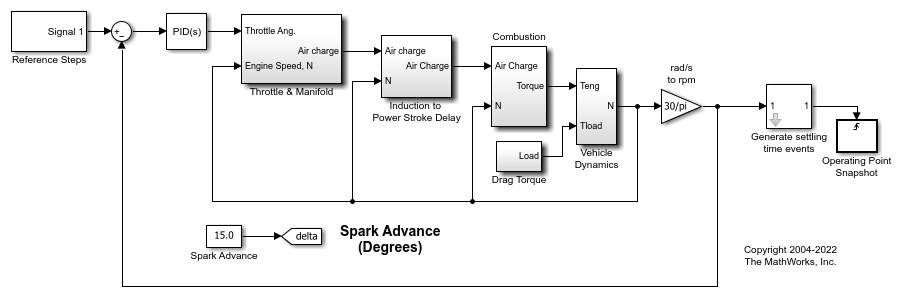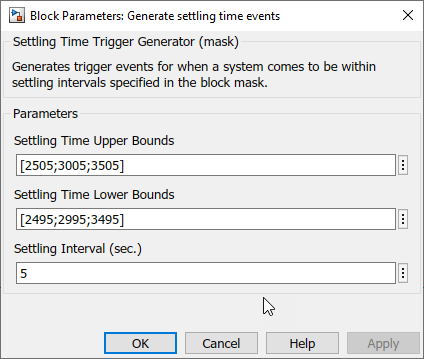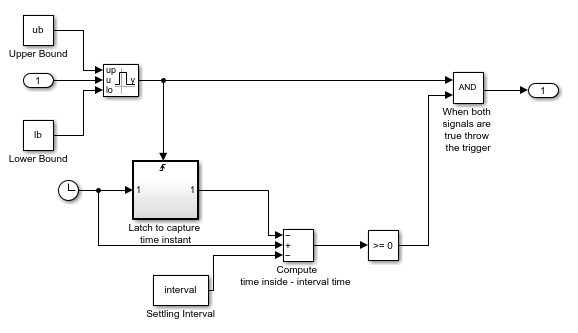Compute Operating Point Snapshots at Triggered Events

This example shows how to generate operating points using triggered simulation snapshots.

Open Model

The model for this example is a speed control system.

Open the model.

mdl = "scdspeedtrigger";
open_system(mdl)The Reference Steps block generates a reference signal that steps through three steady-state speed conditions: 2500, 3000, and 3500 rpm. In this example, you find operating points at each of these conditions by taking operating point snapshots.

Configure Settling Time Events

Since the exact time that a system reaches a steady-state condition is not always known, you can configure your model to detect when a steady-state condition occurs and generate corresponding trigger events.

For this example, the Generate settling time events subsystem detects when the speed signal near a steady-state settling point. The block generates a trigger event when the input signal is within a specified region near the settling point for a minimum amount of time.

For this example, you define regions near the three steady-state speed values. Open the block and specify the upper and lower bounds for these ranges to be 5 rpm above and below the steady-state speed values. To do so, set the Settling Time Upper Bounds and Settling Time Lower Bounds parameters.

Also, specify a minimum settling interval of 5 seconds using the Settling Interval parameter.Within the Generate settling time events subsystem:

• When the input signal is within the specified upper and lower bounds, the Interval Test Dynamic block outputs a true signal.

• The Interval Test Dynamic block output changing from false to true triggers a latching mechanism to track how long the signal is true.

• When the signal is true for a specified interval time, the latching mechanism outputs a true signal.

• When the outputs of the Interval Test Dynamic block and the latching mechanism are both true, the output trigger signal is set to true.The trigger signal from the Generate settling time events subsystem connects to a Trigger-Based Operating Point Snapshot block. You can configure this block to take operating point snapshots on the rising or falling edge of a trigger signal. For this example, the block uses the rising edge of the trigger signal.

Find Operating Points

To compute the operating points, use the findop function to simulate the model for 60 seconds. This function returns a vector of four operating points: one for each triggered snapshot time and one at a simulation time of 60 seconds.

op = findop(mdl,60);

The first operating point is near the 2500 rpm (261.8 rad/s) settling condition.

op(1)
ans =

Operating point for the Model scdspeedtrigger.
(Time-Varying Components Evaluated at time t=10.63)

States:
----------
<strong>x</strong>
<strong>_______</strong>

(1.) scdspeedtrigger/PID Controller/Filter/Cont. Filter/Filter
0
(2.) scdspeedtrigger/PID Controller/Integrator/Continuous/Integrator
10.47
(3.) scdspeedtrigger/Throttle & Manifold/Intake Manifold/p0 = 0.543 bar
0.51066
(4.) scdspeedtrigger/Vehicle Dynamics/w = T//J w0 = 209 rad//s
261.8

Inputs: None
----------

The second operating point is near the 3000 rpm (314.16 rad/s) settling condition.

op(2)
ans =

Operating point for the Model scdspeedtrigger.
(Time-Varying Components Evaluated at time t=28.3703)

States:
----------
<strong>x</strong>
<strong>_______</strong>

(1.) scdspeedtrigger/PID Controller/Filter/Cont. Filter/Filter
0
(2.) scdspeedtrigger/PID Controller/Integrator/Continuous/Integrator
11.915
(3.) scdspeedtrigger/Throttle & Manifold/Intake Manifold/p0 = 0.543 bar
0.49012
(4.) scdspeedtrigger/Vehicle Dynamics/w = T//J w0 = 209 rad//s
314.16

Inputs: None
----------

The third operating point is near the 3500 rpm (366.52 rad/s) settling condition.

op(3)
ans =

Operating point for the Model scdspeedtrigger.
(Time-Varying Components Evaluated at time t=48.2688)

States:
----------
<strong>x</strong>
<strong>_______</strong>

(1.) scdspeedtrigger/PID Controller/Filter/Cont. Filter/Filter
0
(2.) scdspeedtrigger/PID Controller/Integrator/Continuous/Integrator
13.349
(3.) scdspeedtrigger/Throttle & Manifold/Intake Manifold/p0 = 0.543 bar
0.47835
(4.) scdspeedtrigger/Vehicle Dynamics/w = T//J w0 = 209 rad//s
366.52

Inputs: None
----------

For an example that linearizes the speed control model at these operating points, see Linearize at Triggered Simulation Events.

bdclose(mdl)

Related Topics